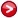# Systematic classification of three-loop realizations of the Weinberg operator: Extra data

In the paper "Ricardo Cepedello, Renato M. Fonseca and Martin Hirsch, Systematic classification of three-loop realizations of the Weinberg operator, arXiv:1807.00629 [hep-ph]"(see also it's erratum) we analyzed all the possible ways of generating the Weinberg operator $HHLL$ via diagrams with three loops.

But in many cases, the fields used to generate a 3-loop diagram will also contribute to neutrino masses through other, more dominant diagrams with less loops. Therefore we used the concept of a genuine 3-loop diagram to refer to those cases which can indeed provide the dominant neutrino mass contribution. One of our main conclusions is that, out of thousands, there are only 499 genuine diagrams, and each is associated to one of 73 topologies. By removing the Higgs VEV insertions from these 499 diagrams, one obtains 38 amputated genuine diagrams.

As explained in our paper, the genuine topologies, diagrams and amputated diagrams were divided into normal and special ones (the latter are only genuine in models with very particular fields).

Due to the large number of diagrams and topologies involved, it was not advisable to present them all in printed form. Nevertheless, they can be found in this page. These files below contains the following data:

1. All 3-loop connected topologies which can be built with 3- and 4-point vertices, containing 4 external lines [allTopologies]
2. The topologies in (I) for which there is at least one diagram yielding the Weinberg operator (2 external fermions + 2 external scalars) using only renormalizable vertices [renormalizableTopologies]
4. The topologies in (III) with no self-energy loops [noSelfEnergyTopologies]
5. The topologies in (IV) which are not disconnected by deletion of one line (i.e. the 1-particle-irreducible ones) [onePITopologies]
6. What we called the "normal genuine topologies" [normalGenuineTopologies]
7. What we called the "special genuine topologies" [specialGenuineTopologies]
8. What we called the "non-genuine finite topologies" [nonGenuineFiniteTopologies]
9. What we called the "non-genuine infinite topologies" [nonGenuineInfiniteTopologies]
10. What we called the "normal genuine diagrams" [normalGenuineDiagrams]]
11. What we called the "special genuine diagrams" [specialGenuineDiagrams]
12. What we called the "amputated normal genuine diagrams", i.e. normal genuine diagrams in the mass basis [normalGenuineAmpDiagrams]
13. What we called the "amputated special genuine diagrams", i.e. the special genuine diagrams in the mass basis [specialGenuineAmpDiagrams]

This data is here:. It has been updated on 04/June/2019 to reflect the problem described in an erratum to the original paper. There are two files in the archive: dataFile with the raw data, and the Mathematica file Weinberg3Loops.nb which loads it and presents some examples. Topologies are saved as adjacency matrices A such that A[[i,j]] contains the number of lines between the vertex i and vertex j. They can be displayed graphically with the built-in function AdjacencyGraph.

Diagrams are also saved as matrices D, where D[[i,j]] is a list indicating what is connecting vertex i to vertex j ("F" stands for a fermion, and an "S" represents a scalar). For example D[[i,j]]={} would mean that the two vertices are not connected, {"S"} would mean that they are connected by a scalar, and {"S","F"} implies that a scalar and a fermion connect them. We have included in dataFile the function PaintLabelledDiagram which can be used to plot diagrams specified in this way.Author
Renato Fonseca

Emails
renatofonseca@gmail.com
or
fonseca@ipnp.mff.cuni.cz

Last updated
08 April 2020